Anzeige

# Data Wrangling and Visualization Using Python

18. Jul 2019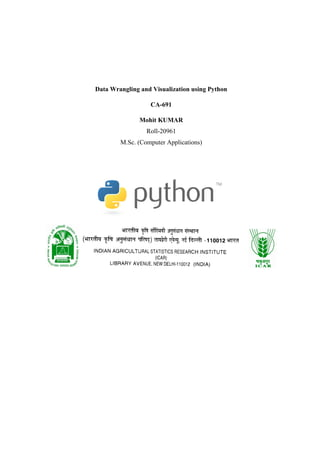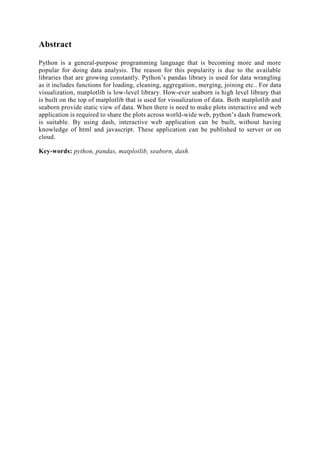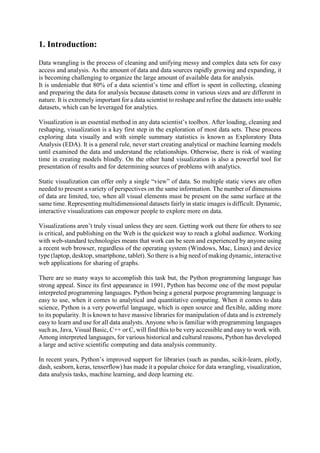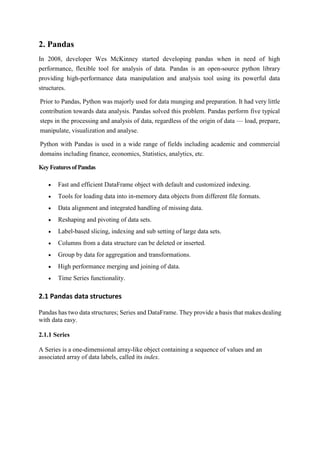Anzeige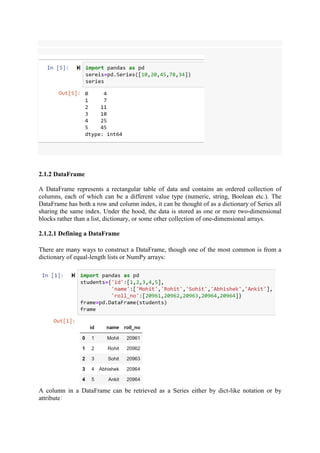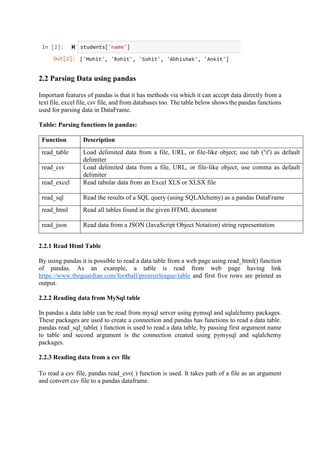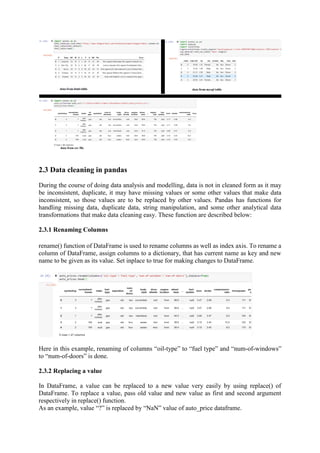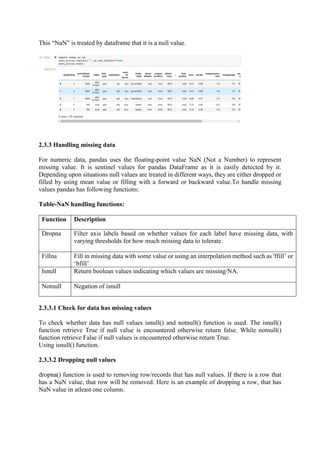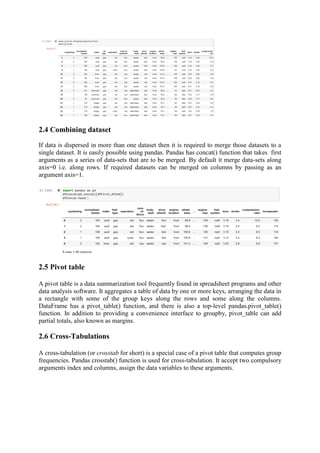Anzeige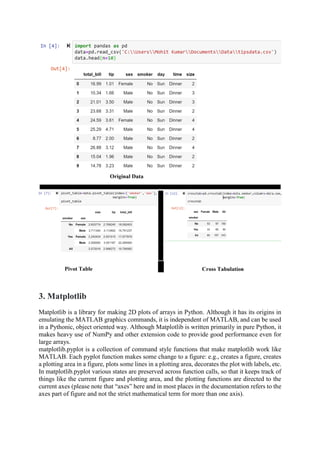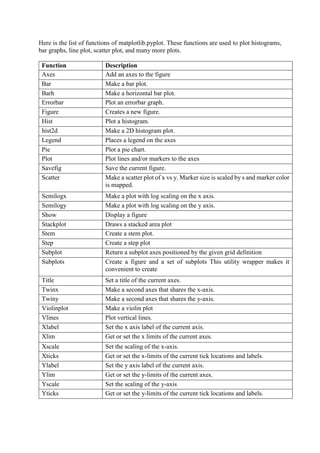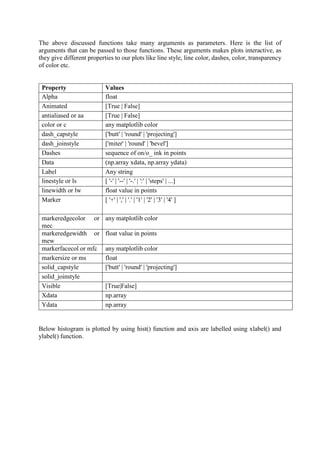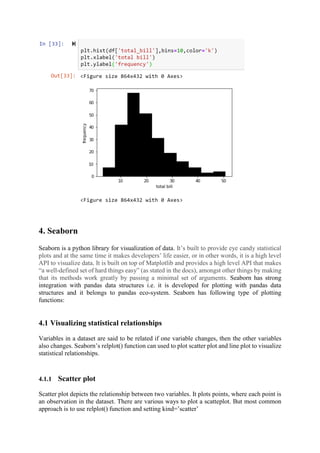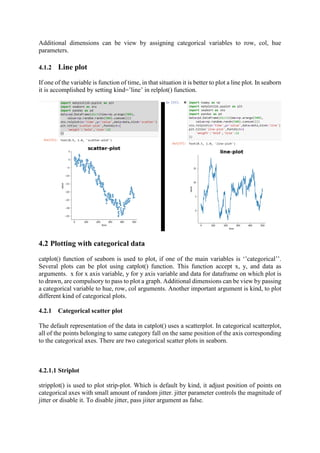Anzeige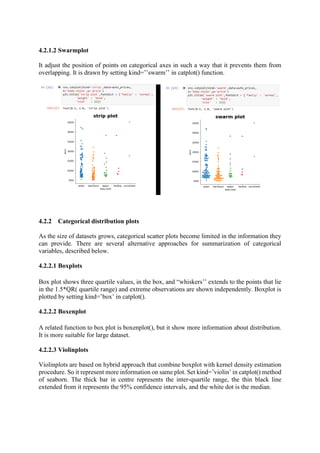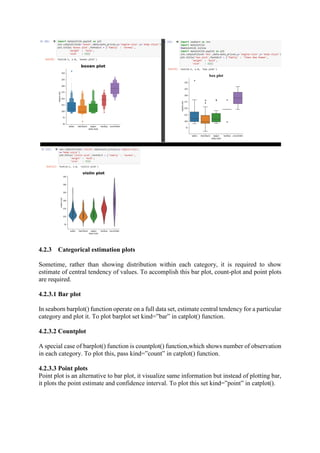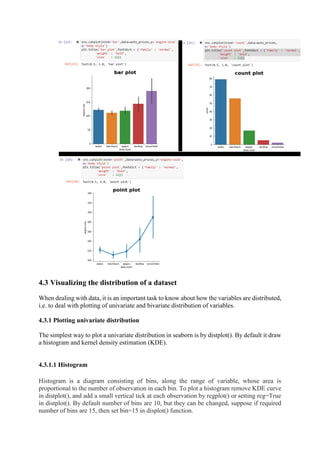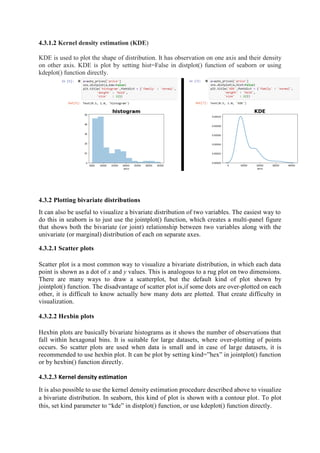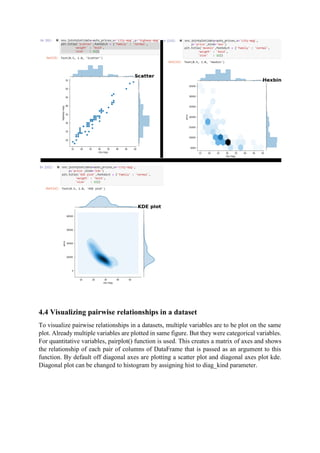Anzeige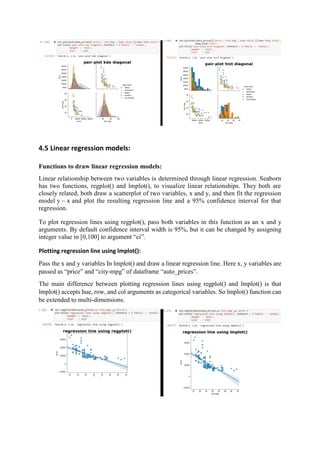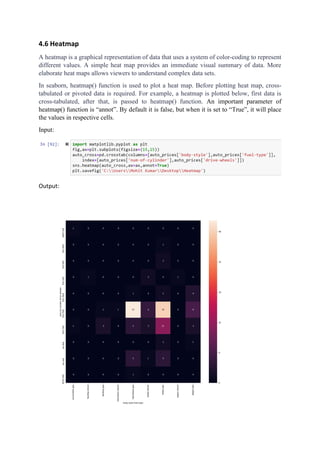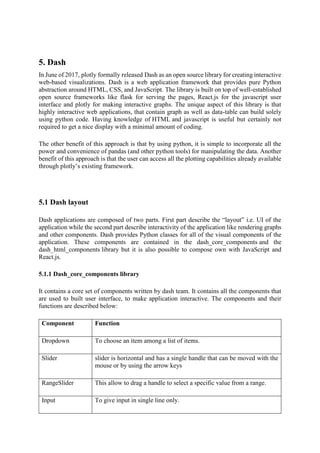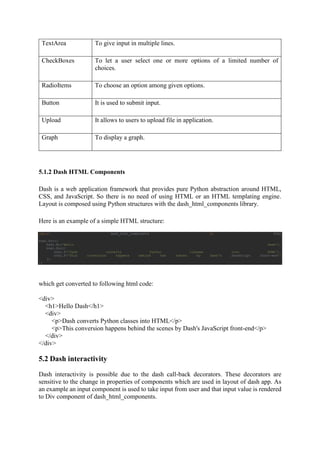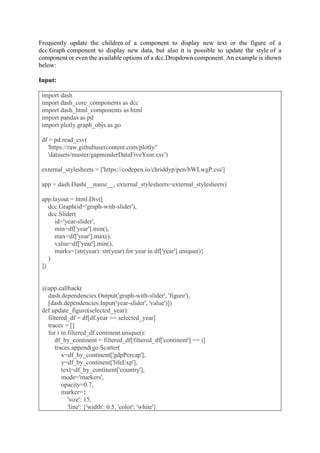Anzeige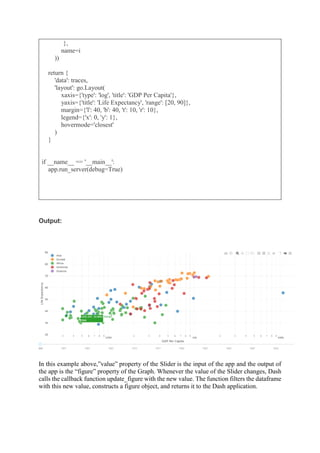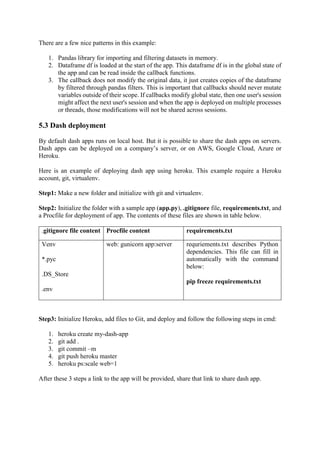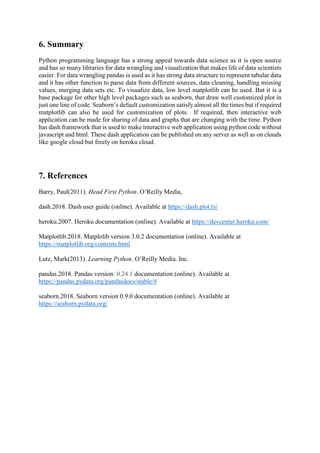Nächste SlideSharePython NumPy Tutorial | NumPy Array | Edureka
1 von 28
Anzeige

### Data Wrangling and Visualization Using Python

1. Data Wrangling and Visualization using Python CA-691 Mohit KUMAR Roll-20961 M.Sc. (Computer Applications)
2. Abstract Python is a general-purpose programming language that is becoming more and more popular for doing data analysis. The reason for this popularity is due to the available libraries that are growing constantly. Python’s pandas library is used for data wrangling as it includes functions for loading, cleaning, aggregation, merging, joining etc.. For data visualization, matplotlib is low-level library. How-ever seaborn is high level library that is built on the top of matplotlib that is used for visualization of data. Both matplotlib and seaborn provide static view of data. When there is need to make plots interactive and web application is required to share the plots across world-wide web, python’s dash framework is suitable. By using dash, interactive web application can be built, without having knowledge of html and javascript. These application can be published to server or on cloud. Key-words: python, pandas, matplotlib, seaborn, dash.
3. 1. Introduction: Data wrangling is the process of cleaning and unifying messy and complex data sets for easy access and analysis. As the amount of data and data sources rapidly growing and expanding, it is becoming challenging to organize the large amount of available data for analysis. It is undeniable that 80% of a data scientist’s time and effort is spent in collecting, cleaning and preparing the data for analysis because datasets come in various sizes and are different in nature. It is extremely important for a data scientist to reshape and refine the datasets into usable datasets, which can be leveraged for analytics. Visualization is an essential method in any data scientist’s toolbox. After loading, cleaning and reshaping, visualization is a key first step in the exploration of most data sets. These process exploring data visually and with simple summary statistics is known as Exploratory Data Analysis (EDA). It is a general rule, never start creating analytical or machine learning models until examined the data and understand the relationships. Otherwise, there is risk of wasting time in creating models blindly. On the other hand visualization is also a powerful tool for presentation of results and for determining sources of problems with analytics. Static visualization can offer only a single “view” of data. So multiple static views are often needed to present a variety of perspectives on the same information. The number of dimensions of data are limited, too, when all visual elements must be present on the same surface at the same time. Representing multidimensional datasets fairly in static images is difficult. Dynamic, interactive visualizations can empower people to explore more on data. Visualizations aren’t truly visual unless they are seen. Getting work out there for others to see is critical, and publishing on the Web is the quickest way to reach a global audience. Working with web-standard technologies means that work can be seen and experienced by anyone using a recent web browser, regardless of the operating system (Windows, Mac, Linux) and device type (laptop, desktop, smartphone, tablet). So there is a big need of making dynamic, interactive web applications for sharing of graphs. There are so many ways to accomplish this task but, the Python programming language has strong appeal. Since its first appearance in 1991, Python has become one of the most popular interpreted programming languages. Python being a general purpose programming language is easy to use, when it comes to analytical and quantitative computing. When it comes to data science, Python is a very powerful language, which is open source and flexible, adding more to its popularity. It is known to have massive libraries for manipulation of data and is extremely easy to learn and use for all data analysts. Anyone who is familiar with programming languages such as, Java, Visual Basic, C++ or C, will find this to be very accessible and easy to work with. Among interpreted languages, for various historical and cultural reasons, Python has developed a large and active scientific computing and data analysis community. In recent years, Python’s improved support for libraries (such as pandas, scikit-learn, plotly, dash, seaborn, keras, tenserflow) has made it a popular choice for data wrangling, visualization, data analysis tasks, machine learning, and deep learning etc.
4. 2. Pandas In 2008, developer Wes McKinney started developing pandas when in need of high performance, flexible tool for analysis of data. Pandas is an open-source python library providing high-performance data manipulation and analysis tool using its powerful data structures. Prior to Pandas, Python was majorly used for data munging and preparation. It had very little contribution towards data analysis. Pandas solved this problem. Pandas perform five typical steps in the processing and analysis of data, regardless of the origin of data — load, prepare, manipulate, visualization and analyse. Python with Pandas is used in a wide range of fields including academic and commercial domains including finance, economics, Statistics, analytics, etc. KeyFeaturesofPandas • Fast and efficient DataFrame object with default and customized indexing. • Tools for loading data into in-memory data objects from different file formats. • Data alignment and integrated handling of missing data. • Reshaping and pivoting of data sets. • Label-based slicing, indexing and sub setting of large data sets. • Columns from a data structure can be deleted or inserted. • Group by data for aggregation and transformations. • High performance merging and joining of data. • Time Series functionality. 2.1 Pandas data structures Pandas has two data structures; Series and DataFrame. They provide a basis that makes dealing with data easy. 2.1.1 Series A Series is a one-dimensional array-like object containing a sequence of values and an associated array of data labels, called its index.
5. 2.1.2 DataFrame A DataFrame represents a rectangular table of data and contains an ordered collection of columns, each of which can be a different value type (numeric, string, Boolean etc.). The DataFrame has both a row and column index, it can be thought of as a dictionary of Series all sharing the same index. Under the hood, the data is stored as one or more two-dimensional blocks rather than a list, dictionary, or some other collection of one-dimensional arrays. 2.1.2.1 Defining a DataFrame There are many ways to construct a DataFrame, though one of the most common is from a dictionary of equal-length lists or NumPy arrays: A column in a DataFrame can be retrieved as a Series either by dict-like notation or by attribute:
7. 2.3 Data cleaning in pandas During the course of doing data analysis and modelling, data is not in cleaned form as it may be inconsistent, duplicate, it may have missing values or some other values that make data inconsistent, so those values are to be replaced by other values. Pandas has functions for handling missing data, duplicate data, string manipulation, and some other analytical data transformations that make data cleaning easy. These function are described below: 2.3.1 Renaming Columns rename() function of DataFrame is used to rename columns as well as index axis. To rename a column of DataFrame, assign columns to a dictionary, that has current name as key and new name to be given as its value. Set inplace to true for making changes to DataFrame. Here in this example, renaming of columns “oil-type” to “fuel type” and “num-of-windows” to “num-of-doors” is done. 2.3.2 Replacing a value In DataFrame, a value can be replaced to a new value very easily by using replace() of DataFrame. To replace a value, pass old value and new value as first and second argument respectively in replace() function. As an example, value “?” is replaced by “NaN” value of auto_price dataframe.
8. This “NaN” is treated by dataframe that it is a null value. 2.3.3 Handling missing data For numeric data, pandas uses the floating-point value NaN (Not a Number) to represent missing value. It is sentinel values for pandas DataFrame as it is easily detected by it. Depending upon situations null values are treated in different ways, they are either dropped or filled by using mean value or filling with a forward or backward value.To handle missing values pandas has following functions: Table-NaN handling functions: Function Description Dropna Filter axis labels based on whether values for each label have missing data, with varying thresholds for how much missing data to tolerate. Fillna Fill in missing data with some value or using an interpolation method such as 'ffill’ or ‘bfill’ Isnull Return boolean values indicating which values are missing/NA. Notnull Negation of isnull 2.3.3.1 Check for data has missing values To check whether data has null values isnull() and notnull() function is used. The isnull() function retrieve True if null value is encountered otherwise return false. While notnull() function retrieve False if null values is encountered otherwise return True. Using isnull() function. 2.3.3.2 Dropping null values dropna() function is used to removing row/records that has null values. If there is a row that has a NaN value, that row will be removed. Here is an example of dropping a row, that has NaN value in atleast one column.
9. 2.4 Combining dataset If data is dispersed in more than one dataset then it is required to merge those datasets to a single dataset. It is easily possible using pandas. Pandas has concat() function that takes first arguments as a series of data-sets that are to be merged. By default it merge data-sets along axis=0 i.e. along rows. If required datasets can be merged on columns by passing as an argument axis=1. 2.5 Pivot table A pivot table is a data summarization tool frequently found in spreadsheet programs and other data analysis software. It aggregates a table of data by one or more keys, arranging the data in a rectangle with some of the group keys along the rows and some along the columns. DataFrame has a pivot_table() function, and there is also a top-level pandas.pivot_table() function. In addition to providing a convenience interface to groupby, pivot_table can add partial totals, also known as margins. 2.6 Cross-Tabulations A cross-tabulation (or crosstab for short) is a special case of a pivot table that computes group frequencies. Pandas crosstab() function is used for cross-tabulation. It accept two compulsory arguments index and columns, assign the data variables to these arguments.
10. 3. Matplotlib Matplotlib is a library for making 2D plots of arrays in Python. Although it has its origins in emulating the MATLAB graphics commands, it is independent of MATLAB, and can be used in a Pythonic, object oriented way. Although Matplotlib is written primarily in pure Python, it makes heavy use of NumPy and other extension code to provide good performance even for large arrays. matplotlib.pyplot is a collection of command style functions that make matplotlib work like MATLAB. Each pyplot function makes some change to a figure: e.g., creates a figure, creates a plotting area in a figure, plots some lines in a plotting area, decorates the plot with labels, etc. In matplotlib.pyplot various states are preserved across function calls, so that it keeps track of things like the current figure and plotting area, and the plotting functions are directed to the current axes (please note that “axes” here and in most places in the documentation refers to the axes part of figure and not the strict mathematical term for more than one axis).
11. Here is the list of functions of matplotlib.pyplot. These functions are used to plot histograms, bar graphs, line plot, scatter plot, and many more plots. Function Description Axes Add an axes to the figure Bar Make a bar plot. Barh Make a horizontal bar plot. Errorbar Plot an errorbar graph. Figure Creates a new figure. Hist Plot a histogram. hist2d Make a 2D histogram plot. Legend Places a legend on the axes Pie Plot a pie chart. Plot Plot lines and/or markers to the axes Savefig Save the current figure. Scatter Make a scatter plot of x vs y. Marker size is scaled by s and marker color is mapped. Semilogx Make a plot with log scaling on the x axis. Semilogy Make a plot with log scaling on the y axis. Show Display a figure Stackplot Draws a stacked area plot Stem Create a stem plot. Step Create a step plot Subplot Return a subplot axes positioned by the given grid definition Subplots Create a figure and a set of subplots This utility wrapper makes it convenient to create Title Set a title of the current axes. Twinx Make a second axes that shares the x-axis. Twiny Make a second axes that shares the y-axis. Violinplot Make a violin plot Vlines Plot vertical lines. Xlabel Set the x axis label of the current axis. Xlim Get or set the x limits of the current axes. Xscale Set the scaling of the x-axis. Xticks Get or set the x-limits of the current tick locations and labels. Ylabel Set the y axis label of the current axis. Ylim Get or set the y-limits of the current axes. Yscale Set the scaling of the y-axis Yticks Get or set the y-limits of the current tick locations and labels.
12. The above discussed functions take many arguments as parameters. Here is the list of arguments that can be passed to those functions. These arguments makes plots interactive, as they give different properties to our plots like line style, line color, dashes, color, transparency of color etc. Property Values Alpha float Animated [True | False] antialiased or aa [True | False] color or c any matplotlib color dash_capstyle ['butt' | 'round' | 'projecting'] dash_joinstyle ['miter' | 'round' | 'bevel'] Dashes sequence of on/o_ ink in points Data (np.array xdata, np.array ydata) Label Any string linestyle or ls [ '-' | '--' | '-.' | ':' | 'steps' | ...] linewidth or lw float value in points Marker [ '+' | ',' | '.' | '1' | '2' | '3' | '4' ] markeredgecolor or mec any matplotlib color markeredgewidth or mew float value in points markerfacecol or mfc any matplotlib color markersize or ms float solid_capstyle ['butt' | 'round' | 'projecting'] solid_joinstyle Visible [True|False] Xdata np.array Ydata np.array Below histogram is plotted by using hist() function and axis are labelled using xlabel() and ylabel() function.
13. 4. Seaborn Seaborn is a python library for visualization of data. It’s built to provide eye candy statistical plots and at the same time it makes developers’ life easier, or in other words, it is a high level API to visualize data. It is built on top of Matplotlib and provides a high level API that makes “a well-defined set of hard things easy” (as stated in the docs), amongst other things by making that its methods work greatly by passing a minimal set of arguments. Seaborn has strong integration with pandas data structures i.e. it is developed for plotting with pandas data structures and it belongs to pandas eco-system. Seaborn has following type of plotting functions: 4.1 Visualizing statistical relationships Variables in a dataset are said to be related if one variable changes, then the other variables also changes. Seaborn’s relplot() function can used to plot scatter plot and line plot to visualize statistical relationships. 4.1.1 Scatter plot Scatter plot depicts the relationship between two variables. It plots points, where each point is an observation in the dataset. There are various ways to plot a scatteplot. But most common approach is to use relplot() function and setting kind=’scatter’
14. Additional dimensions can be view by assigning categorical variables to row, col, hue parameters. 4.1.2 Line plot If one of the variable is function of time, in that situation it is better to plot a line plot. In seaborn it is accomplished by setting kind=’line’ in relplot() function. 4.2 Plotting with categorical data catplot() function of seaborn is used to plot, if one of the main variables is ‘’categorical’’. Several plots can be plot using catplot() function. This function accept x, y, and data as arguments. x for x axis variable, y for y axis variable and data for dataframe on which plot is to drawn, are compulsory to pass to plot a graph. Additional dimensions can be view by passing a categorical variable to hue, row, col arguments. Another important argument is kind, to plot different kind of categorical plots. 4.2.1 Categorical scatter plot The default representation of the data in catplot() uses a scatterplot. In categorical scatterplot, all of the points belonging to same category fall on the same position of the axis corresponding to the categorical axes. There are two categorical scatter plots in seaborn. 4.2.1.1 Striplot stripplot() is used to plot strip-plot. Which is default by kind, it adjust position of points on categorical axes with small amount of random jitter. jitter parameter controls the magnitude of jitter or disable it. To disable jitter, pass jiiter argument as false.
15. 4.2.1.2 Swarmplot It adjust the position of points on categorical axes in such a way that it prevents them from overlapping. It is drawn by setting kind=’’swarm’’ in catplot() function. 4.2.2 Categorical distribution plots As the size of datasets grows, categorical scatter plots become limited in the information they can provide. There are several alternative approaches for summarization of categorical variables, described below. 4.2.2.1 Boxplots Box plot shows three quartile values, in the box, and “whiskers’’ extends to the points that lie in the 1.5*QR( quartile range) and extreme observations are shown independently. Boxplot is plotted by setting kind=’box’ in catplot(). 4.2.2.2 Boxenplot A related function to box plot is boxenplot(), but it show more information about distribution. It is more suitable for large dataset. 4.2.2.3 Violinplots Violinplots are based on hybrid approach that combine boxplot with kernel density estimation procedure. So it represent more information on same plot. Set kind=’violin’ in catplot() method of seaborn. The thick bar in centre represents the inter-quartile range, the thin black line extended from it represents the 95% confidence intervals, and the white dot is the median.
16. 4.2.3 Categorical estimation plots Sometime, rather than showing distribution within each category, it is required to show estimate of central tendency of values. To accomplish this bar plot, count-plot and point plots are required. 4.2.3.1 Bar plot In seaborn barplot() function operate on a full data set, estimate central tendency for a particular category and plot it. To plot barplot set kind=”bar” in catplot() function. 4.2.3.2 Countplot A special case of barplot() function is countplot() function,which shows number of observation in each category. To plot this, pass kind=”count” in catplot() function. 4.2.3.3 Point plots Point plot is an alternative to bar plot, it visualize same information but instead of plotting bar, it plots the point estimate and confidence interval. To plot this set kind=”point” in catplot().
17. 4.3 Visualizing the distribution of a dataset When dealing with data, it is an important task to know about how the variables are distributed, i.e. to deal with plotting of univariate and bivariate distribution of variables. 4.3.1 Plotting univariate distribution The simplest way to plot a univariate distribution in seaborn is by distplot(). By default it draw a histogram and kernel density estimation (KDE). 4.3.1.1 Histogram Histogram is a diagram consisting of bins, along the range of variable, whose area is proportional to the number of observation in each bin. To plot a histogram remove KDE curve in distplot(), and add a small vertical tick at each observation by regplot() or setting reg=True in distplot(). By default number of bins are 10, but they can be changed, suppose if required number of bins are 15, then set bin=15 in displot() function.
18. 4.3.1.2 Kernel density estimation (KDE) KDE is used to plot the shape of distribution. It has observation on one axis and their density on other axis. KDE is plot by setting hist=False in distplot() function of seaborn or using kdeplot() function directly. 4.3.2 Plotting bivariate distributions It can also be useful to visualize a bivariate distribution of two variables. The easiest way to do this in seaborn is to just use the jointplot() function, which creates a multi-panel figure that shows both the bivariate (or joint) relationship between two variables along with the univariate (or marginal) distribution of each on separate axes. 4.3.2.1 Scatter plots Scatter plot is a most common way to visualize a bivariate distribution, in which each data point is shown as a dot of x and y values. This is analogous to a rug plot on two dimensions. There are many ways to draw a scatterplot, but the default kind of plot shown by jointplot() function. The disadvantage of scatter plot is,if some dots are over-plotted on each other, it is difficult to know actually how many dots are plotted. That create difficulty in visualization. 4.3.2.2 Hexbin plots Hexbin plots are basically bivariate histograms as it shows the number of observations that fall within hexagonal bins. It is suitable for large datasets, where over-plotting of points occurs. So scatter plots are used when data is small and in case of large datasets, it is recommended to use hexbin plot. It can be plot by setting kind=”hex” in jointplot() function or by hexbin() function directly. 4.3.2.3 Kernel density estimation It is also possible to use the kernel density estimation procedure described above to visualize a bivariate distribution. In seaborn, this kind of plot is shown with a contour plot. To plot this, set kind parameter to “kde” in distplot() function, or use kdeplot() function directly.
19. 4.4 Visualizing pairwise relationships in a dataset To visualize pairwise relationships in a datasets, multiple variables are to be plot on the same plot. Already multiple variables are plotted in same figure. But they were categorical variables. For quantitative variables, pairplot() function is used. This creates a matrix of axes and shows the relationship of each pair of columns of DataFrame that is passed as an argument to this function. By default off diagonal axes are plotting a scatter plot and diagonal axes plot kde. Diagonal plot can be changed to histogram by assigning hist to diag_kind parameter.
20. 4.5 Linear regression models: Functions to draw linear regression models: Linear relationship between two variables is determined through linear regression. Seaborn has two functions, regplot() and lmplot(), to visualize linear relationships. They both are closely related, both draw a scatterplot of two variables, x and y, and then fit the regression model y ~ x and plot the resulting regression line and a 95% confidence interval for that regression. To plot regression lines using regplot(), pass both variables in this function as an x and y arguments. By default confidence interval width is 95%, but it can be changed by assigning integer value in [0,100] to argument “ci”. Plotting regression line using lmplot(): Pass the x and y variables In lmplot() and draw a linear regression line. Here x, y variables are passed as “price” and “city-mpg” of dataframe “auto_prices”. The main difference between plotting regression lines using regplot() and lmplot() is that lmplot() accepts hue, row, and col arguments as categorical variables. So lmplot() function can be extended to multi-dimensions.
21. 4.6 Heatmap A heatmap is a graphical representation of data that uses a system of color-coding to represent different values. A simple heat map provides an immediate visual summary of data. More elaborate heat maps allows viewers to understand complex data sets. In seaborn, heatmap() function is used to plot a heat map. Before plotting heat map, cross- tabulated or pivoted data is required. For example, a heatmap is plotted below, first data is cross-tabulated, after that, is passed to heatmap() function. An important parameter of heatmap() function is “annot”. By default it is false, but when it is set to “True”, it will place the values in respective cells. Input: Output:
22. 5. Dash In June of 2017, plotly formally released Dash as an open source library for creating interactive web-based visualizations. Dash is a web application framework that provides pure Python abstraction around HTML, CSS, and JavaScript. The library is built on top of well-established open source frameworks like flask for serving the pages, React.js for the javascript user interface and plotly for making interactive graphs. The unique aspect of this library is that highly interactive web applications, that contain graph as well as data-table can build solely using python code. Having knowledge of HTML and javascript is useful but certainly not required to get a nice display with a minimal amount of coding. The other benefit of this approach is that by using python, it is simple to incorporate all the power and convenience of pandas (and other python tools) for manipulating the data. Another benefit of this approach is that the user can access all the plotting capabilities already available through plotly’s existing framework. 5.1 Dash layout Dash applications are composed of two parts. First part describe the “layout” i.e. UI of the application while the second part describe interactivity of the application like rendering graphs and other components. Dash provides Python classes for all of the visual components of the application. These components are contained in the dash_core_components and the dash_html_components library but it is also possible to compose own with JavaScript and React.js. 5.1.1 Dash_core_components library It contains a core set of components written by dash team. It contains all the components that are used to built user interface, to make application interactive. The components and their functions are described below: Component Function Dropdown To choose an item among a list of items. Slider slider is horizontal and has a single handle that can be moved with the mouse or by using the arrow keys RangeSlider This allow to drag a handle to select a specific value from a range. Input To give input in single line only.
23. TextArea To give input in multiple lines. CheckBoxes To let a user select one or more options of a limited number of choices. RadioItems To choose an option among given options. Button It is used to submit input. Upload It allows to users to upload file in application. Graph To display a graph. 5.1.2 Dash HTML Components Dash is a web application framework that provides pure Python abstraction around HTML, CSS, and JavaScript. So there is no need of using HTML or an HTML templating engine. Layout is composed using Python structures with the dash_html_components library. Here is an example of a simple HTML structure: import dash_html_components as html html.Div([ html.H1('Hello Dash'), html.Div([ html.P('Dash converts Python classes into HTML'), html.P('This conversion happens behind the scenes by Dash's JavaScript front-end') ]) ]) which get converted to following html code: <div> <h1>Hello Dash</h1> <div> <p>Dash converts Python classes into HTML</p> <p>This conversion happens behind the scenes by Dash's JavaScript front-end</p> </div> </div> 5.2 Dash interactivity Dash interactivity is possible due to the dash call-back decorators. These decorators are sensitive to the change in properties of components which are used in layout of dash app. As an example an input component is used to take input from user and that input value is rendered to Div component of dash_html_components.
24. Frequently update the children of a component to display new text or the figure of a dcc.Graph component to display new data, but also it is possible to update the style of a component or even the available options of a dcc.Dropdown component. An example is shown below: Input: import dash import dash_core_components as dcc import dash_html_components as html import pandas as pd import plotly.graph_objs as go df = pd.read_csv( 'https://raw.githubusercontent.com/plotly/' 'datasets/master/gapminderDataFiveYear.csv') external_stylesheets = ['https://codepen.io/chriddyp/pen/bWLwgP.css'] app = dash.Dash(__name__, external_stylesheets=external_stylesheets) app.layout = html.Div([ dcc.Graph(id='graph-with-slider'), dcc.Slider( id='year-slider', min=df['year'].min(), max=df['year'].max(), value=df['year'].min(), marks={str(year): str(year) for year in df['year'].unique()} ) ]) @app.callback( dash.dependencies.Output('graph-with-slider', 'figure'), [dash.dependencies.Input('year-slider', 'value')]) def update_figure(selected_year): filtered_df = df[df.year == selected_year] traces = [] for i in filtered_df.continent.unique(): df_by_continent = filtered_df[filtered_df['continent'] == i] traces.append(go.Scatter( x=df_by_continent['gdpPercap'], y=df_by_continent['lifeExp'], text=df_by_continent['country'], mode='markers', opacity=0.7, marker={ 'size': 15, 'line': {'width': 0.5, 'color': 'white'}
25. }, name=i )) return { 'data': traces, 'layout': go.Layout( xaxis={'type': 'log', 'title': 'GDP Per Capita'}, yaxis={'title': 'Life Expectancy', 'range': [20, 90]}, margin={'l': 40, 'b': 40, 't': 10, 'r': 10}, legend={'x': 0, 'y': 1}, hovermode='closest' ) } if __name__ == '__main__': app.run_server(debug=True) Output: In this example above,”value” property of the Slider is the input of the app and the output of the app is the “figure” property of the Graph. Whenever the value of the Slider changes, Dash calls the callback function update_figure with the new value. The function filters the dataframe with this new value, constructs a figure object, and returns it to the Dash application.
26. There are a few nice patterns in this example: 1. Pandas library for importing and filtering datasets in memory. 2. Dataframe df is loaded at the start of the app. This dataframe df is in the global state of the app and can be read inside the callback functions. 3. The callback does not modify the original data, it just creates copies of the dataframe by filtered through pandas filters. This is important that callbacks should never mutate variables outside of their scope. If callbacks modify global state, then one user's session might affect the next user's session and when the app is deployed on multiple processes or threads, those modifications will not be shared across sessions. 5.3 Dash deployment By default dash apps runs on local host. But it is possible to share the dash apps on servers. Dash apps can be deployed on a company’s server, or on AWS, Google Cloud, Azure or Heroku. Here is an example of deploying dash app using heroku. This example require a Heroku account, git, virtualenv. Step1: Make a new folder and initialize with git and virtualenv. Step2: Initialize the folder with a sample app (app.py), .gitignore file, requirements.txt, and a Procfile for deployment of app. The contents of these files are shown in table below. .gitignore file content Procfile content requirements.txt Venv *.pyc .DS_Store .env web: gunicorn app:server requriements.txt describes Python dependencies. This file can fill in automatically with the command below: pip freeze requirements.txt Step3: Initialize Heroku, add files to Git, and deploy and follow the following steps in cmd: 1. heroku create my-dash-app 2. git add . 3. git commit –m 4. git push heroku master 5. heroku ps:scale web=1 After these 3 steps a link to the app will be provided, share that link to share dash app.
27. 6. Summary Python programming language has a strong appeal towards data science as it is open source and has so many libraries for data wrangling and visualization that makes life of data scientists easier. For data wrangling pandas is used as it has strong data structure to represent tabular data and it has other function to parse data from different sources, data cleaning, handling missing values, merging data sets etc. To visualize data, low level matplotlib can be used. But it is a base package for other high level packages such as seaborn, that draw well customized plot in just one line of code. Seaborn’s default customization satisfy almost all the times but if required matplotlib can also be used for customization of plots. If required, then interactive web application can be made for sharing of data and graphs that are changing with the time. Python has dash framework that is used to make interactive web application using python code without javascript and html. These dash application can be published on any server as well as on clouds like google cloud but freely on heroku cloud. 7. References Barry, Paul(2011). Head First Python. O’Reilly Media, dash.2018. Dash user guide (online). Available at https://dash.plot.ly/ heroku.2007. Heroku documentation (online). Available at https://devcenter.heroku.com/ Matplotlib.2018. Matplolib version 3.0.2 documentation (online). Available at https://matplotlib.org/contents.html Lutz, Mark(2013). Learning Python. O’Reilly Media. Inc. pandas.2018. Pandas version: 0.24.1 documentation (online). Available at https://pandas.pydata.org/pandasdocs/stable/# seaborn.2018. Seaborn version 0.9.0 documentation (online). Available at https://seaborn.pydata.org/
Anzeige